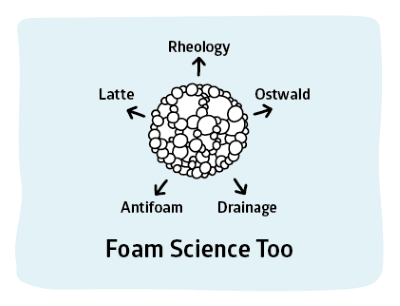## Foam Rheology

### Quick Start

Foam rheology is a specialised area. But it's not entirely obscure. If you make a shaving foam or a firefighting foam, how it behaves when you try to make it move is really rather important. Fortunately, the app is easy to use.

The inputs are the bubble radius, the surface tension, the fraction of air, φ, and the viscosity of the bulk fluid (typically 1 cP). You get a modulus and yield stress and a couple of graphs about what happens to stress and viscosity at different shear rates.

### Foam Rheology

SurfTen γ mN/m
Air Fraction φ
Viscosity μ cP
Modulus G Pa
Yield Stress Y Pa
Surface DissipationRheology is an immense and subtle subject and the complexities of foams make it even harder to grasp. Because this is Practical Foams we'll concentrate on a few key factors that directly impact day-to-day foam work. The formulae are taken from the Rheology chapter by Denkov et al in Stevenson's Foam Engineering book1. The factors are:

• Modulus (how elastic the foam is)
• Yield Stress (how resistant it is to movement)
• Viscosity under shear (viscosity changing with velocity and gap of flow)

Not surprisingly, but happily, the factors over which we have direct control have the biggest effects on the rheology:

• Surface tension γ
• Air fraction Φ in the region of 0.7-0.99, with some fudging at the lower end.

### Modulus G

With some uncertainty around the lower values of Φ which are not of practical interest

G=(1.4Φ(Φ-0.64)γ)/R

### Yield Stress

With the same uncertainty around the lower values of Φ

Y=(0.5(Φ-0.64)γ)/R

### Viscosity and shear

Ignoring (for simplicity) wall-induced viscosity, there are two different forms of viscous dissipation:

• τVF Sliding friction (bubbles sliding past bubbles)
• τVS Surface dissipation (bubbles expand/contract during motion and dissipate energy)

As discussed especially in Drainage, some co-surfactants such as fatty acids or alcohols can migrate to the surface and form a highly rigid surface. It is these types of foam (represented by the standard "Gillette" foam) which show the second type of dissipation. Simple ("Dawn" type) foams are dominated by the sliding friction.

It can be shown elegantly that these effects can be well-modelled by the sorts of non-dimensional analyses (rightly) loved by academics. But Practical people need dimensional numbers so the equations shown (and implemented) are inelegant but useful. For these equations we need the bulk viscosity µ and shear rate S. Of course shear rate is usually designated as a dotted gamma, but because we are using γ for surface tension, S seems more practical.

τ_"VF"=1.16(γ/R)((μSR)/γ)^0.47(Φ^(5/6)(Φ-0.74)^0.1)/(sqrt(1-Φ))

τ_"VS"=9.8(γ/R)Φ^2BS

The factor B in the second equation is so far not readily calculated. It varies from 0 (no surface dissipation) to maybe 3E-3. In this app a value of 2E-3 (which has been experimentally determined in a typical "Gillette" foam) is applied when the Surface Dissipation option is selected. Academics tend to plot stress v. shear, users tend to prefer viscosity v. shear. Both are plotted for convenience. Moving the mouse over the graph gives values converted from the log plot. In the stress plot the theoretical stress curve plus the more realistic one that includes yield stress are shown. Because stresses less than the yield stress are not meaningful for viscosity for that plot the curve is shown only for shears that give a stress higher than the yield

The take-home message from all this is that foams with small bubbles are stronger, more resistant and somewhat more shear thinning than those with large bubbles. Also drier foams are stronger etc. That's intuitively obvious, but it's good to have numbers to back up the intuitions.

1Nikolai Denkov et al, Foam Rheology, Ch 6 in Paul Stevenson (Ed) Foam Engineering: Fundamentals and Applications, Wiley, 2012